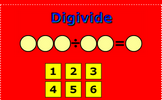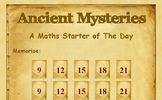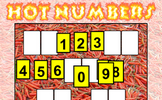# Developing number sense

### Term 3 starting in week 7 :: Estimated time: 2 weeks

• Know and use mental addition and subtraction strategies for integers
• Know and use mental multiplication and division strategies for integers
• Know and use mental arithmetic strategies for decimals
• Know and use mental arithmetic strategies for fractions
• Use factors to simplify calculations
• Use estimation as a method for checking mental calculations
• Use known number facts to derive other facts
• Use known algebraic facts to derive other facts
• Know when to use a mental strategy, formal written method or a calculator

This page should remember your ticks from one visit to the next for a period of time. It does this by using Local Storage so the information is saved only on the computer you are working on right now.

## Lesson Starters

Here are some suggestions for whole-class, projectable resources which can be used at the beginnings of each lesson in this block.

### 1st Lesson#### Digivide

Arrange the digits 1 to 6 to make a three digit number divided by a two digit number giving a one digit answer.

### 2nd Lesson#### Ancient Mysteries

This activity requires students to memorise fifteen numbers in a three by five grid.

### 3rd Lesson#### Half and Half

Start with 100. Halve it to get 50. Halve that to get 25. Continue as far as possible.

### 4th Lesson#### Hot Numbers

Move the numbered cards to form five 2 digit numbers which are all multiples of three.

### 5th Lesson#### Countdown

How close can you get to the target by making a calculation from the five numbers given?

### 6th Lesson#### Kim's Game 1

This is the Maths version of the traditional memory game. Memorise 10 mathematical facts then recall them as they disappear from view.

Some of the Starters above are to reinforce concepts learnt, others are to introduce new ideas while others are on unrelated topics designed for retrieval practice or and opportunity to develop problem-solving skills.

White Rose ResourcesEnd of block assessments provide a quick progress check at the end of each block of learning to make sure students have understood the content covered. This Scheme of Learning was produced by White Rose Maths and is used here with permission granted on 30th June 2021.For All: